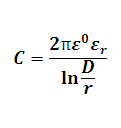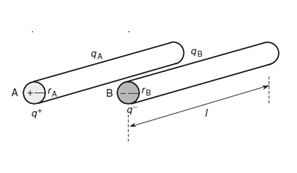# Capacitance of Single Phase Line Transmission Calculator

This Calctown Calculator calculates the capacitance of a single phase transmission line.

unit
m
m

#### Result

FClick here to view image

where

ε0 = Dielectric constant in vaccum

εr = Dielectric ratio

D = distance between the lines

r = radius of conductors

C = Capacitance